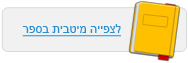עמוד:29 4 . A child is swinging on a swing . A . In the following table , identify the types of energy for each position ( A , B , C ) . B . In an energy flow diagram , describe the energy changes that occur during this process . 5 . Specify one appliance that you use . Using the following table , describe the energy changes that take place in it , using both words and an energy diagram . See the example . 6 . Describe an example that corresponds to each of the following energy diagrams . a . Electrical energy heat . b . Potential energy kinetic energy . 7 . Give an example that corresponds to each of the following flow charts ( the example can be an imaginary one ) . a . Radiation energy electrical energy kinetic energy b . Potential energy kinetic energy electrical energy heat Position C - The girl is at the maximum height on the right side . Position B – The girl is at the lowest point in the midlle Position A - The girl is at the maximum height on the left side .© מטח - המרכז לטכנולוגיה חינוכית